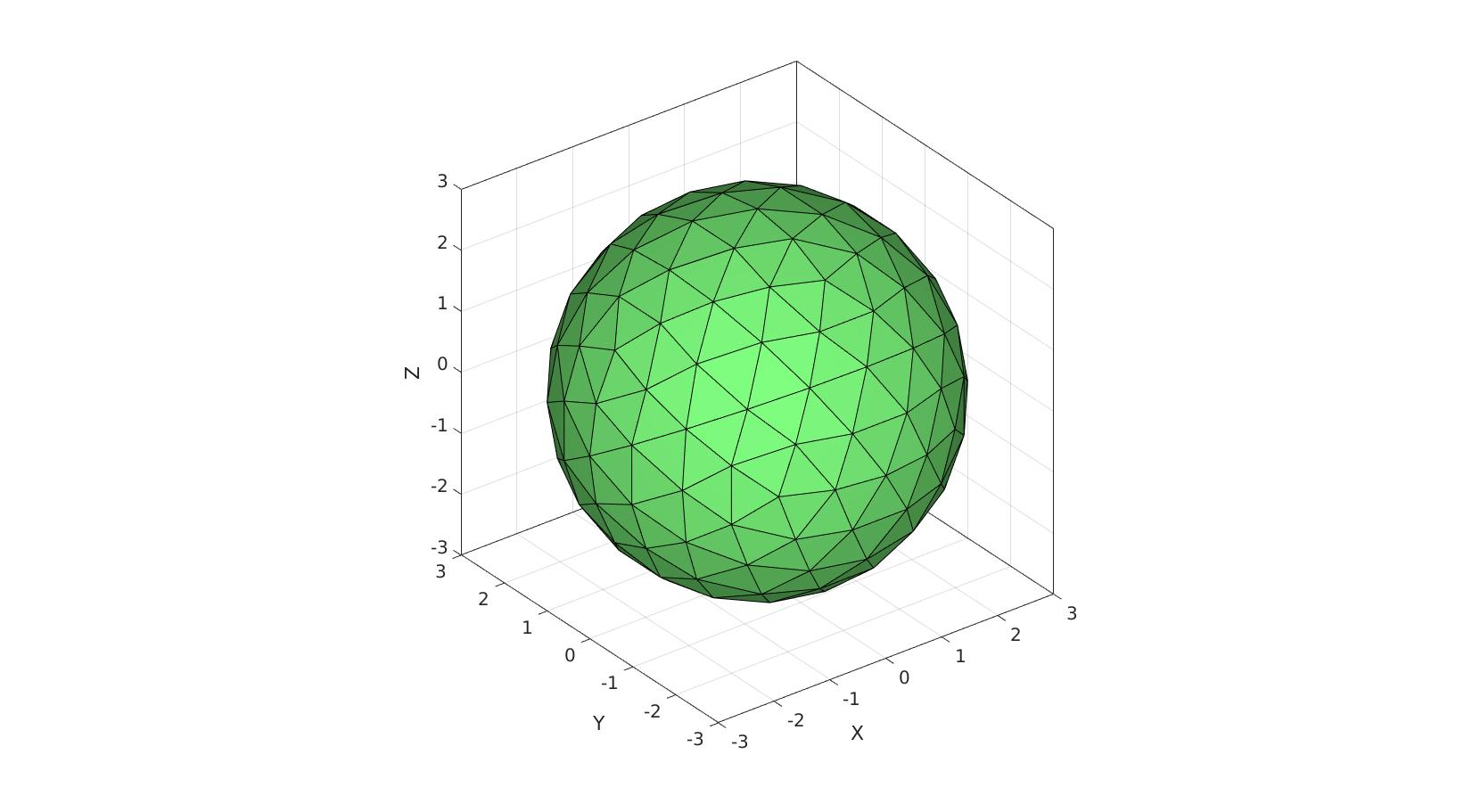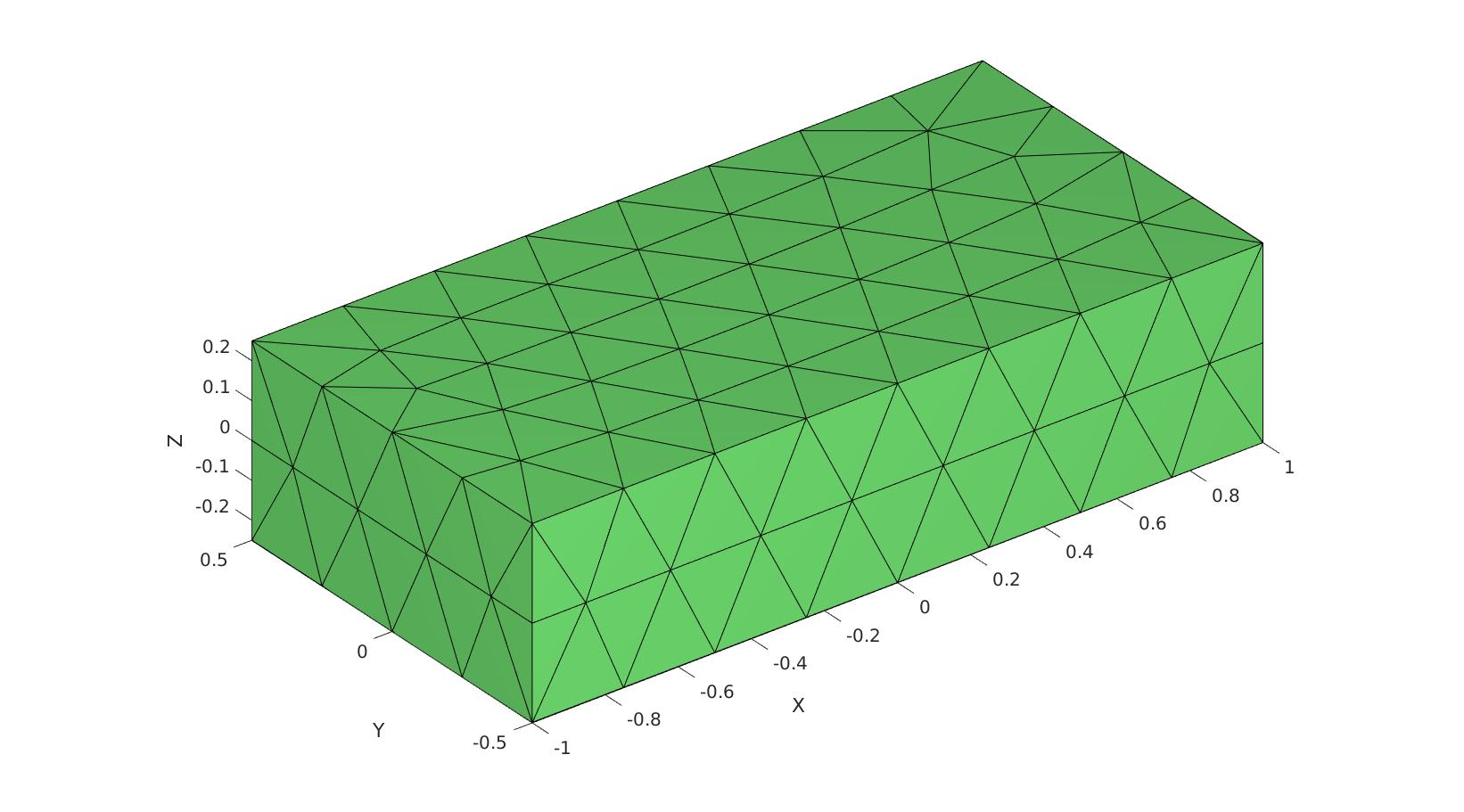# triSurfVolume

Below is a demonstration of the features of the triSurfVolume function

## Contents

```clear; close all; clc;
```

## Syntax

[surfaceVolume]=triSurfVolume(F,V);

UNDOCUMENTED

## Example 1: Volume of a sphere

Creating meshed sphere, note that the discrete nature of the mesh causes the volume to deviate somewhat from the theoretical value.

```r=3; %sphere radius
n=2; %Refinements
[F,V,~]=geoSphere(n,r);
```

Computing the volume

```vol_theoretical=(4/3)*pi*r.^3 %Theoretical volume
vol_surf_est=triSurfVolume(F,V) %estimate based on triangulated surface
```
```vol_theoretical =

113.0973

vol_surf_est =

109.2702

```
```cFigure;
gpatch(F,V,'gw','k');
gdrawnow;
```## Example 2: Volume of a beam

Creating a meshed beam

```% Specifying dimensions and number of elements for each direction
boxDim=[2 1 0.5]; %Width in each direction
pointSpacing=min(boxDim)/2; %Desired point spacing
[F,V,faceBoundaryMarker]=triBox(boxDim,pointSpacing); % Using |triBox| to build a triangulated cube
```Computing the volume

```vol_theoretical=prod(boxDim) %Theoretical volume
vol_surf_est=triSurfVolume(F,V) %estimate based on triangulated surface
```
```vol_theoretical =

1

vol_surf_est =

1.0000

```
```cFigure;
gpatch(F,V,'gw','k');
gdrawnow;
```GIBBON www.gibboncode.org

Kevin Mattheus Moerman, [email protected]

GIBBON footer text

GIBBON: The Geometry and Image-based Bioengineering add-On. A toolbox for image segmentation, image-based modeling, meshing, and finite element analysis.

Copyright (C) 2006-2022 Kevin Mattheus Moerman and the GIBBON contributors

This program is free software: you can redistribute it and/or modify it under the terms of the GNU General Public License as published by the Free Software Foundation, either version 3 of the License, or (at your option) any later version.

This program is distributed in the hope that it will be useful, but WITHOUT ANY WARRANTY; without even the implied warranty of MERCHANTABILITY or FITNESS FOR A PARTICULAR PURPOSE. See the GNU General Public License for more details.

You should have received a copy of the GNU General Public License along with this program. If not, see http://www.gnu.org/licenses/.